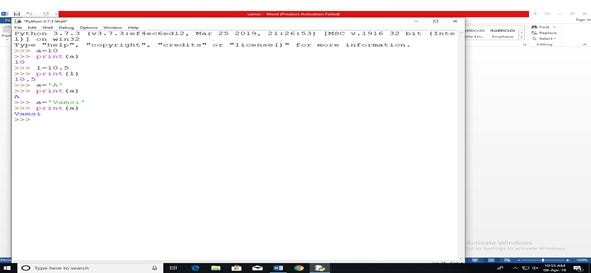Our Special Offer - Get 3 Courses at 24,999/- Only. Read more
Hire Talent (HR):+91-9707 240 250

# General# Python Input and Output

### Python Input:

Input is nothing but a data given to the console to start a task. There are so many types of inputs are there in python.

There is no difference of input either in python 2x to the python 3x version. Whatever it may be the version the way of accessing or utilizing the data is the same.

#### INPUT

Input is the function which is use for inputting the data that can store in the variable (OR) It is a keyword used to collect the data from the user.

### Types:

• Input
• Raw-input

Syntax:

```Variable name =input()
V_n=input(‘Statement’)```

Example:

` a=input(‘Enter ur value’)`

Note: By default, the output of input is a string even though if you assign the other format of data also it will convert that to the string.

### The other way of representing the input:

Syntax:

` V_n=data type(input(‘Statement’))`

Example:

` A=int (input (‘Enter your data’))`

### Python Input and Output### Python Input and Output ExampleExample input function

```a=int(input(“enter the integer value”))
b=float(input(“enter the integer value”))
print(type(a))
print(type(b))```

Output

```10
10.55
C:\Users\My Name>python demo_numbers.py
<class 'int'>
<class 'float'>.```

### Python Input and Output Function

Python being a cross-platform programming language can be easily run on multiple platforms like Windows, MacOS, and Linux and has even been ported to the Java and .NET virtual machines. It is an open source that is free to use.

• Number
• String
• Collection

#### Number

There are 3 numeric types in Python

#### Int:

int (plain integers): this one is pretty standard — plain integers are just positive or negative whole numbers

Syntax:

`Variable name = data type`

Example:

```a=10 #int
print(a)#output function```

example:

```b=10.33 #float
c=  1j #complex variable
print(b)#output function
print(c) #output function```

#### String :

Sting is a collection of variable it stores the chart.

Syntax:

`Sting variable: collection of chart`

Example:

```name=input(“enter the name”)#taking the input from the keyboard
Print(name) #output function```

Example:

```name=”karthik”#default string variable
Print(name) #output function
```